blog

# Weighted Moving Average: What Is It And How Do You Use It?

#### Options Trading 101 - The Ultimate Beginners Guide To Options

As Seen On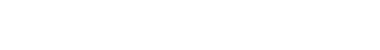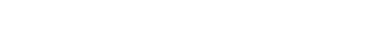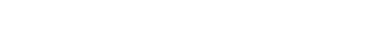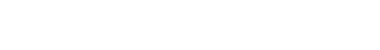by Gavin in Blog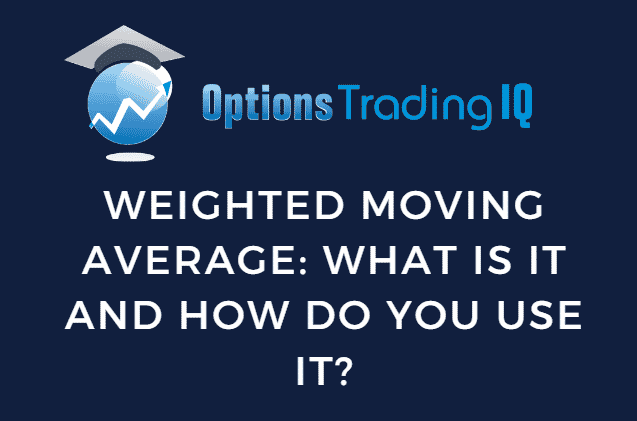## What Is A Weighted Moving Average?

A weighted moving average can be an extremely useful resource for investors because it prioritizes certain portions of the data.

This can ultimately help investors with tough trading decisions and help them make smart decisions when trading in the market.

Usually, a weighted moving average will allow recent data to overtake some data that might be slightly more outdated from a charting pattern.

While a weighted moving average doesn’t completely ignore the older data on the chart, it simply prioritizes more of the recent data on the chart to create an average that will ultimately determine momentum, trend, and volatility.

Since a weighted moving average prioritizes recent data, it is more likely for the real-time price to remain close to the weighted moving average.

When an investment’s real-time price drastically shifts in any direction away from the average, it could signal to place a contrarian order to take advantage of the short-term volatility.

Experienced investors may be able to take advantage of a weighted moving average as a resource for trading.

Still, it should certainly not be the only indicator to rely on when making trading decisions.

It’s always a good idea to use multiple indicators in addition to the moving average before finalizing a trade decision.

By using multiple indicators, you are building a general consensus about the market you plan on trading in, which can help prevent bad decisions or trading mistakes in the long run.

## How Do You Calculate A Weighted Moving Average?

According to the Fidelity brokerage, this is a solid example of a formula that could be used to calculate a weighted moving average. (Source)

Weighted Moving Average Formula

WMA = (P1 * 5) + (P2 * 4) + (P3 * 3) + (P4 * 2) + (P5 * 1) / (5 + 4 + 3 + 2 + 1)

P1 = Real-Time Price

P2 = Last Candlestick

P3 = Two Candlesticks Ago

P4 = Three Candlesticks Ago

P5 = Four Candlesticks Ago

The formula demonstrates how the real-time price is multiplied by a higher number than outdated candlesticks.

This difference in weight gets more significant as the candlesticks get older and older until you reach the beginning of the timeframe that you are calculating the weighted moving average.

This type of calculation can be modified by adjusting the weighted formula that is being used.

There are many different types of weighted moving averages, and the formula can be adjusted based on the type of investment that you are making.

A traditional moving average won’t be as volatile, while a weighted moving average will stay fairly close to the real-time price.

Some investors believe that regions of support or resistance can be identified by using a formula to calculate the weighted moving average.

In addition, early indications of a bearish or bullish market trend can be identified by experienced investors familiar with the weighted moving average calculation.

While the weighted moving average formula can be extremely helpful for determining the current trend of an investment, it isn’t always reliable.

In fact, no charting indicators are correct 100% of the time.

## Weighted Moving Average Example

Let’s pretend that we’re measuring the last four days of the closing price of a particular stock.

For this example, let’s pretend the stock price closed at \$25 on day one, \$20 on day two, \$30 on day three, and \$40 on the final and most recent day.

Day 1 =  10% of the weight moving average.

Day 2  = 20% of the weight moving average.

Day 3 =  30% of the weight moving average.

Day 4 =  40% of the weight moving average.

WMA = [\$40 x (4/10)] + [\$30 x (3/10)] + [\$20 x (2/10)] + [25 x (1/10)]

Every investor has a different idea of how they want their weighted moving average to work.

Some people prefer having way more weight leaning towards recent data on charts, while others may prefer keeping older data relevant.

Long-term investors may be more willing to keep weight leaning towards older data, while short-term day traders and swing traders may prefer something different.

You should be encouraged to play around and look at different ways to adjust your weighted moving average formula to find something that works for the type of trades you plan on making.

Experienced investors are always making changes to their moving average formulas.

Sometimes, it can even be useful to rely on multiple weighted moving average formulas and compare them over time to see which has been more reliable and helpful.

## How Do Traders Use The Weighted Moving Average?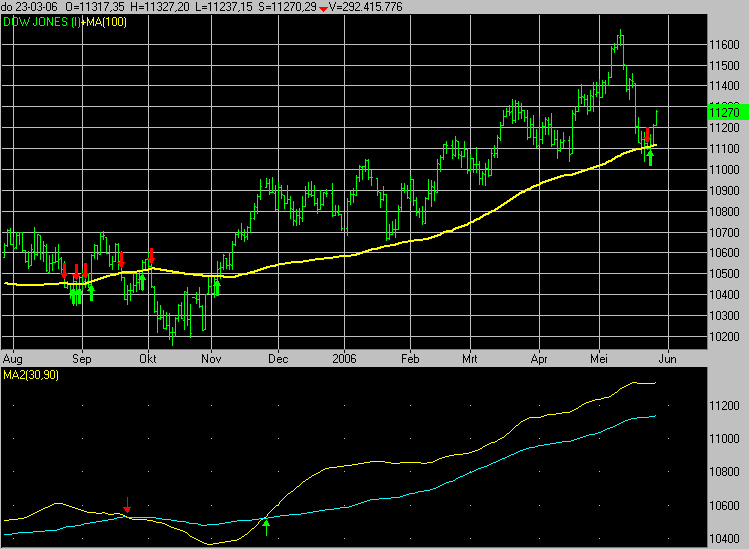Weighted moving averages can be an exceptional resource for investors, but don’t be completely fooled.

There is a higher chance of receiving a false trading signal if you rely on a weighted moving average as your primary charting indicator.

Let’s take a look at some reasons a trader or investor may want to rely on a weighted moving average.

Swing traders are always looking for signals of a potential dip in the market.

A weighted moving average may be able to draw a baseline on a horizontal real-time trading chart that can help send buy signals when a stock drops below the weighted moving average.

Depending on how much you rely on recent data, there are some instances where this type of calculation can be extremely helpful and effective.

There are also times when a weighted moving average can trick investors into making an investment based on a false signal.

In addition to trying to detect buy and sell signals for future trades, the weighted moving average can be used as a companion indicator with other common charting resources to find support and resistance areas on a chart.

Determining a particular investment’s overall trend and momentum is also a great effective way to take advantage of the weighted moving average.

Short-term traders generally use the weighted moving average, and that is the type of investor that will find the most use out of the weighted moving average.

While it’s possible for long-term investors to also use the weighted moving average, their trading strategies will rely less on the weighted moving average because recent charting data doesn’t always have an impact on trading decisions relating to investors that have strategies based on the long haul.

## Simple Moving Average vs. Weighted Moving Average

The simple moving average is slightly different from the weighted moving average because it focuses on the generalized average price over a certain period.

For example, let’s assume the time frame that we are measuring is one year.

A simple moving average will average the price of an investment over the entire year, while a weighted moving average will take all of the same information but place way more weight on more recent data points.

The simple moving average is way easier to calculate because you can simply take the closing price of a particular investment every single trading day of the year and add all of those prices up before dividing the sum against the total number of trading days per year.

Here’s an example of a simple moving average formula over four days.

Day 1 =  25% of the weight moving average.

Day 2  = 25% of the weight moving average.

Day 3 =  25% of the weight moving average.

Day 4 = 25% of the weight moving average.

All four days will have an equal amount of weight applied to the simple moving average, indicating that no particular day will have more impact than any other day.

Day 1 = \$25.00 Closing Price

Day 2 = \$28.00 Closing Price

Day 3 = \$26.00 Closing Price

Day 4 = \$31.00 Closing Price

Now that we have placed some example closing prices on each day let’s demonstrate how we would use this information to calculate the simple moving average for our imaginary scenario.

SMA = 25 + 28 + 26 + 31 / 4 = \$27.50 (Simple Moving Average)

A weighted moving average would be a little bit different because it would heavily rely on recent data, placing the highest weight on the fourth and final day while slowly placing less weight as you get further into the past.

## FAQ

### How do you add a moving average to a chart?

Almost all free and premium charting software will have moving averages available as an indicator.

If you want to add a custom moving average to a chart, simply determine the weight you want to place on recent data and select a timeframe.

Once you have inputted this information, your moving average should appear on the chart.

You can also conduct the calculation the old-fashioned way and get a result that way, but that is not always ideal for investors nowadays due to the technological advances that have been made in various trading software.

### Which moving average is best for swing trading?

Short-term moving averages and weighted moving averages are ideal for swing trading because they prioritize recent data, which can be extremely useful for making short-term trading decisions based on momentum and trends.

Investors should always use multiple moving average indicators to cross-check their trading theories before finalizing any trading decision because relying on a single indicator can be risky.

### Which is better, SMA or EMA?

Long-term investors will likely rely more on simple moving averages because all of the data within a given timeframe is weighted equally.

Short-term traders may like exponential moving averages because recent data receive more weight in the calculation.

Both moving averages can be extremely helpful, but it really depends on what type of trades you are planning on taking.

Day and swing traders can rely on exponential and weighted moving averages because they rely on recent short-term data.

Long-term investors might be more confident with the simple moving average because it takes a look at the long-term history of the chart’s trend.

### What happens when the 50-day moving average crosses the 200-day moving average?

Many investors call this indication a ‘death cross.’

This occurs when the 200-day moving average falls below the 50-day moving average, signaling a long-term change in momentum on the chart.

This is usually followed by bearish market conditions for the foreseeable future until the momentum changes the other way.

When the opposite indication occurs, investors call it a ‘golden cross,’ which is signaled when the 50-day moving average crosses above the 200-day long-term moving average.